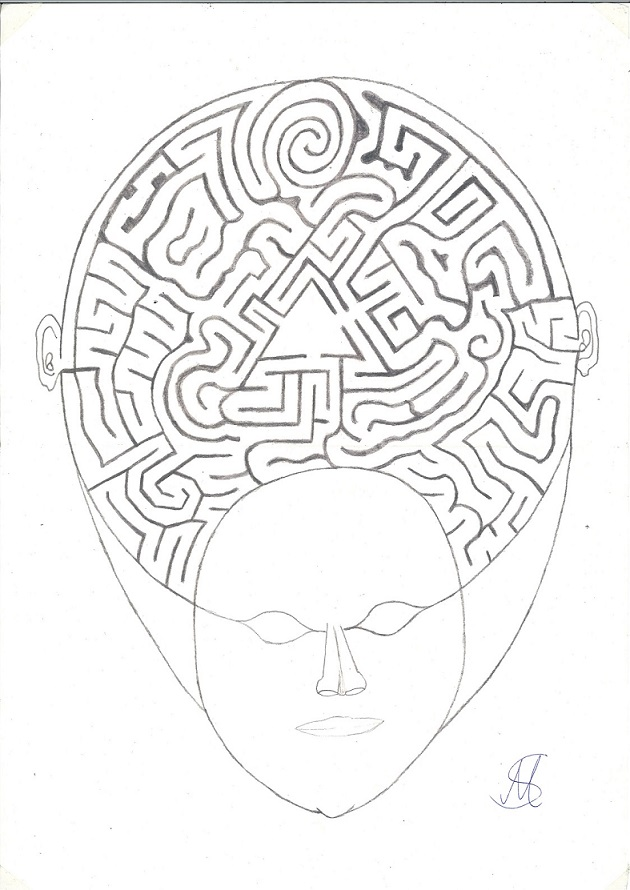# 中文的 (Chinese, Mandarin) 世界状态

## 简历 Markus Schrems.pdf## 空间填充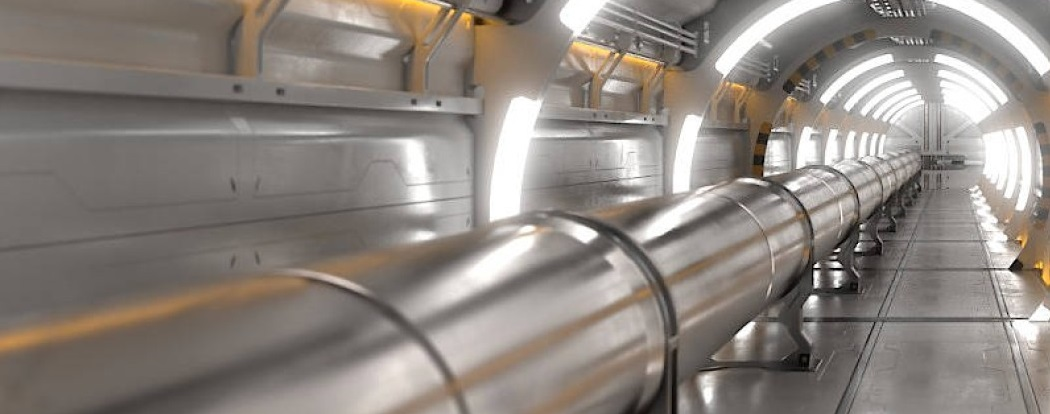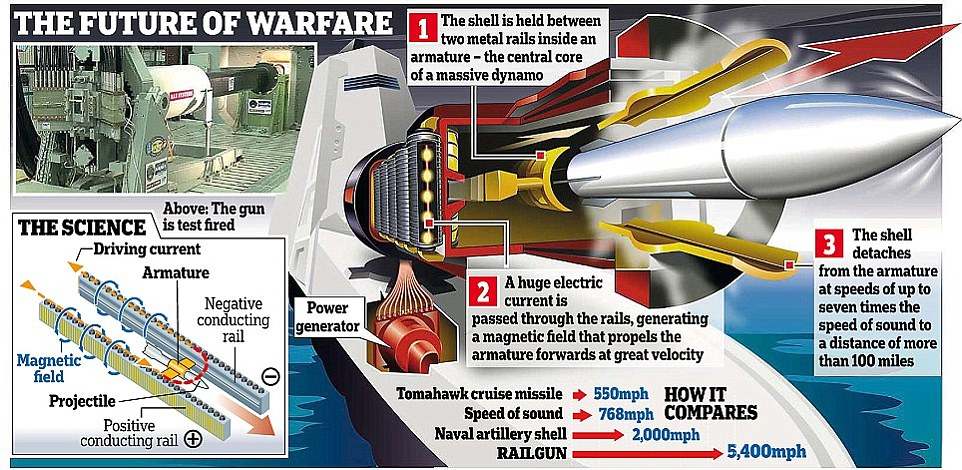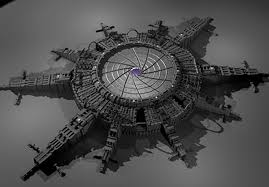v^2 = u^2 + 2as

a = v^2 / (2s)

a = 2780^2 [米/秒] / (2 * 5 [米])

a ≈ 772 840 m/s^2

v^2 = u^2 + 2as

v = √(2as)

v = √(2 * 772 840 * 3 000 000)

v ≈ 2 153 379 m/s = 7 752 163 [km/h]]

Or with the Helical-Engine.

## Or scientist discovered a warp-bubble coincidentally!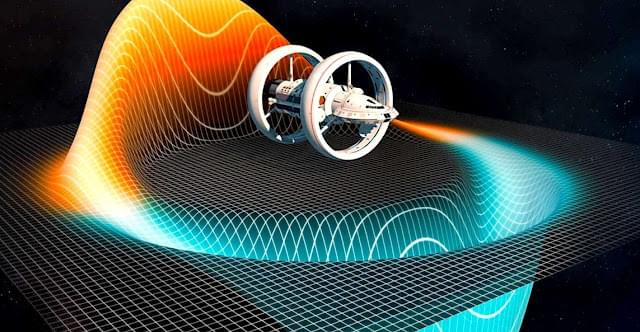Alcubierre-Drive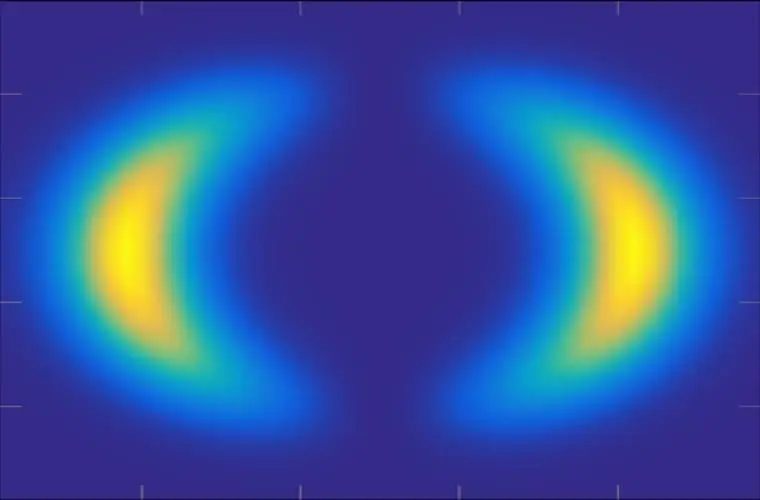Warp-Bubble

## 量子场波动涡轮机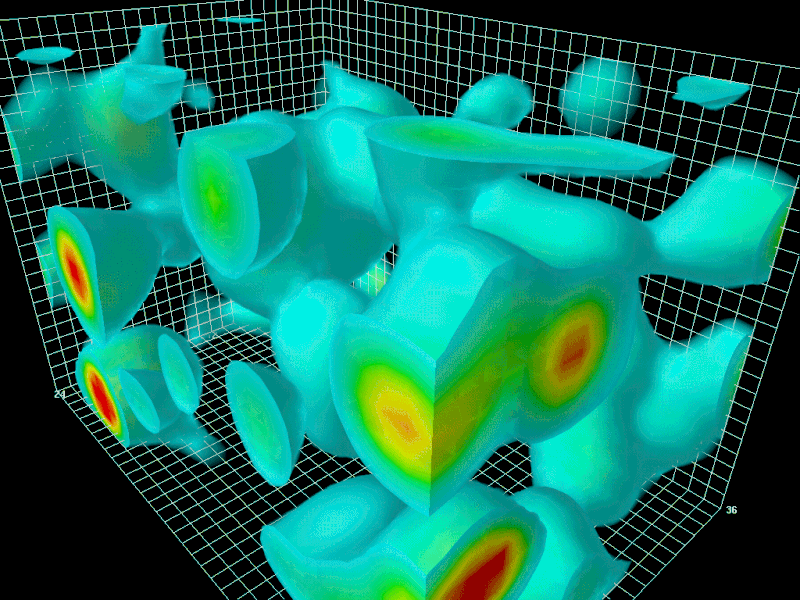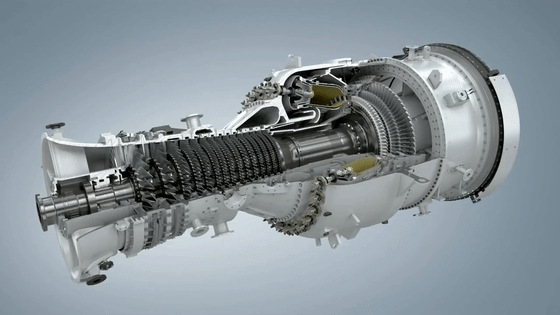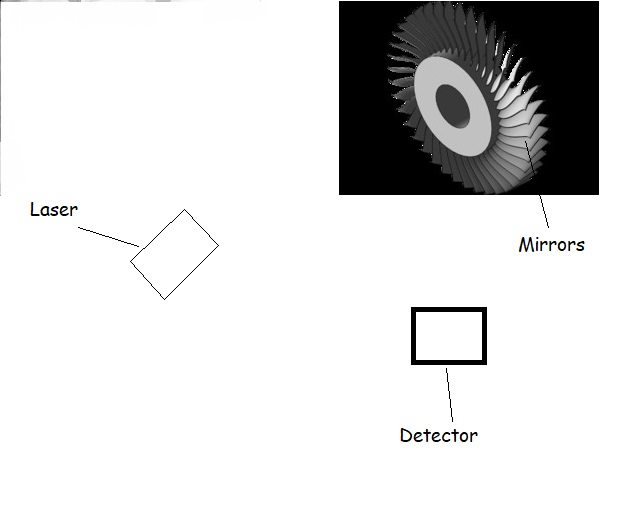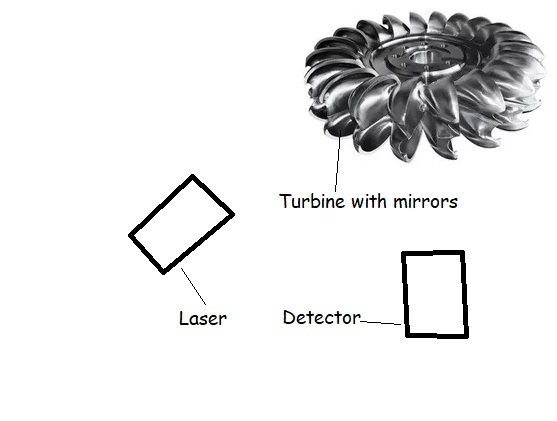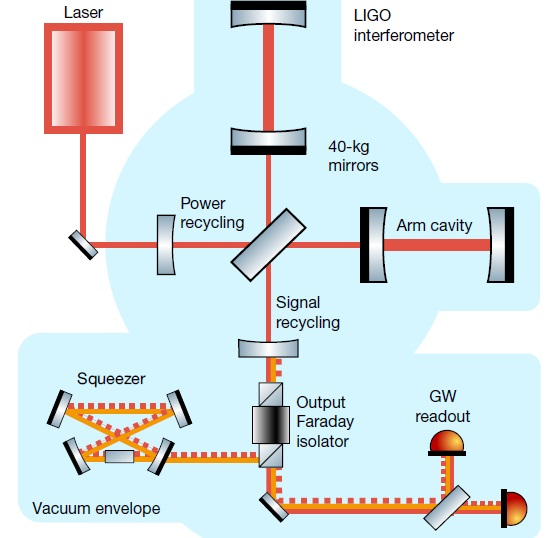p_st 或光压是由于吸收、发射或反射电磁辐射而作用在表面上的压力。 在吸收和发射过程中，辐射压等于波的强度 I 除以光速 c： p_st = I/c，单位为 p_st = N/m2 = J/m3 = Pa（帕斯卡）； 完全反射时的辐射压力是完全吸收时的两倍。 频率为 ν 的光子传输能量： E = h * ν （参见光电效应） 与普朗克常数 h。

Tij = (1/ 2)*( ε_0*E² + B²/ μ_0 )*δij − ε_0*Ei*Ej − Bi*Bj/ μ_0

## 手动手扇卡西米尔效果：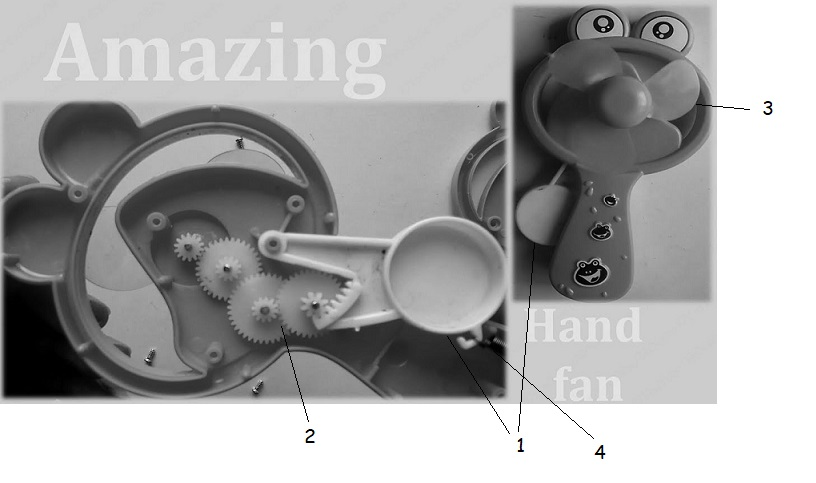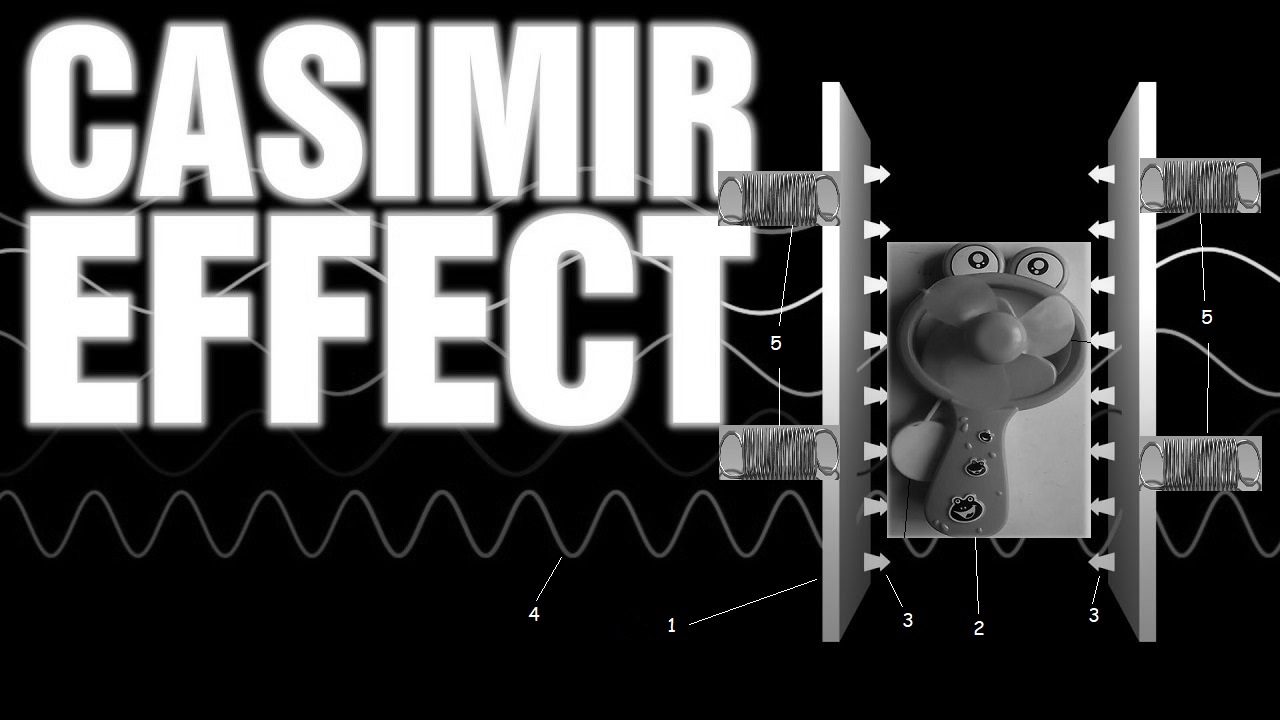## 海鲜农场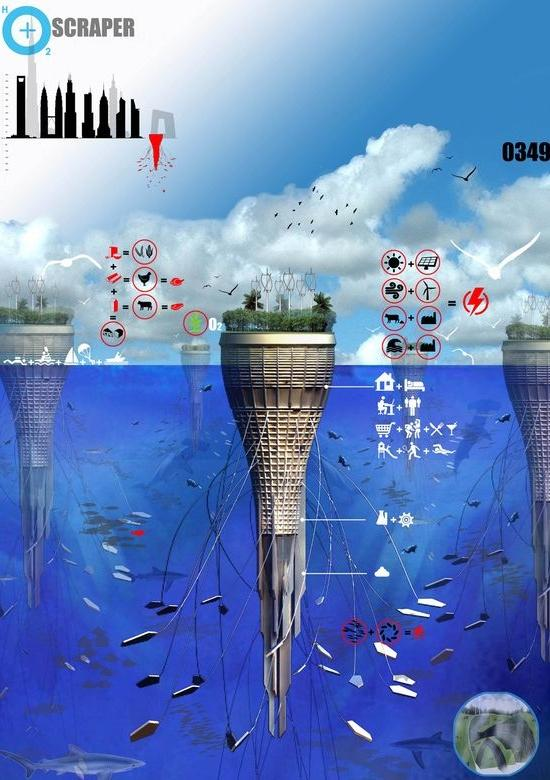## 碳工程网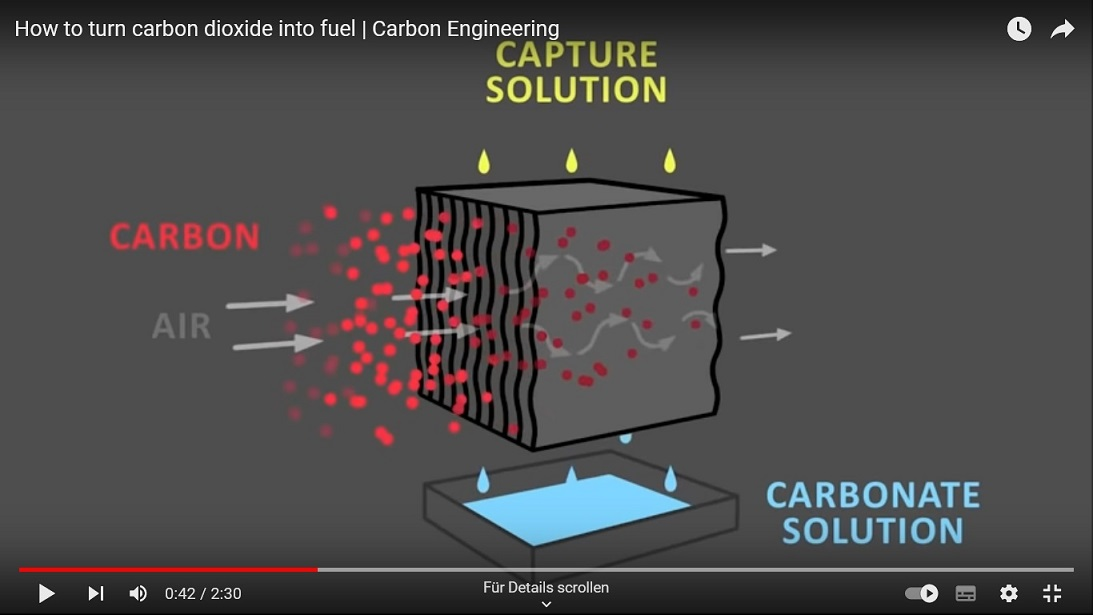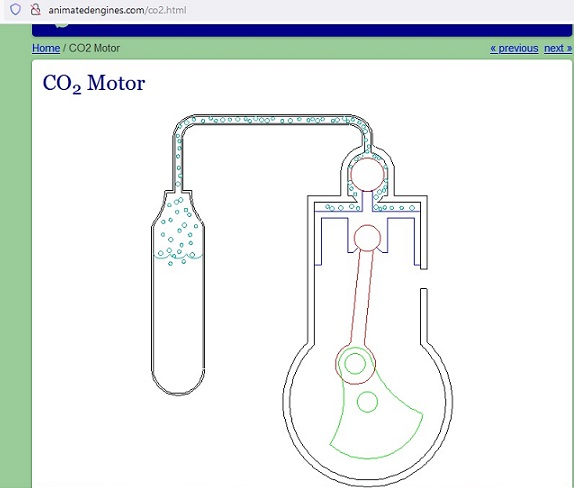CO2-Engine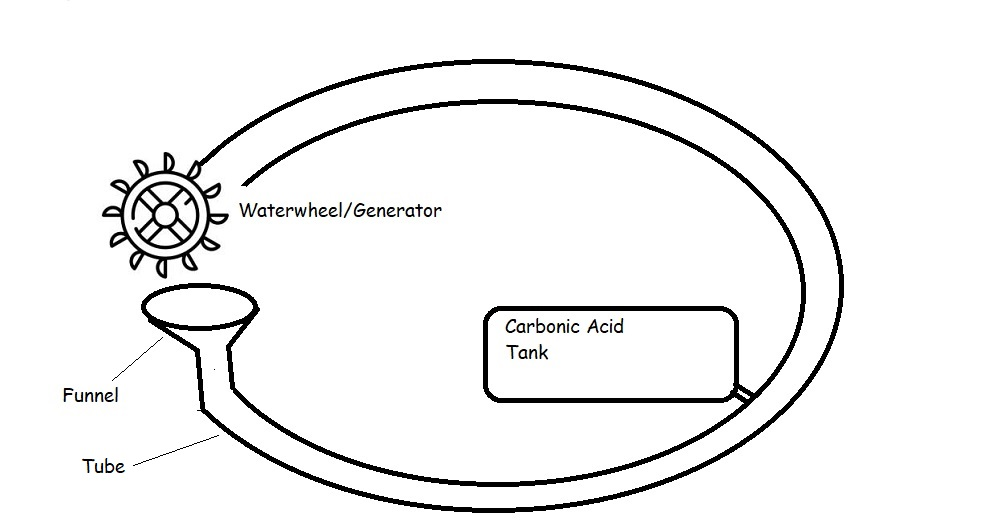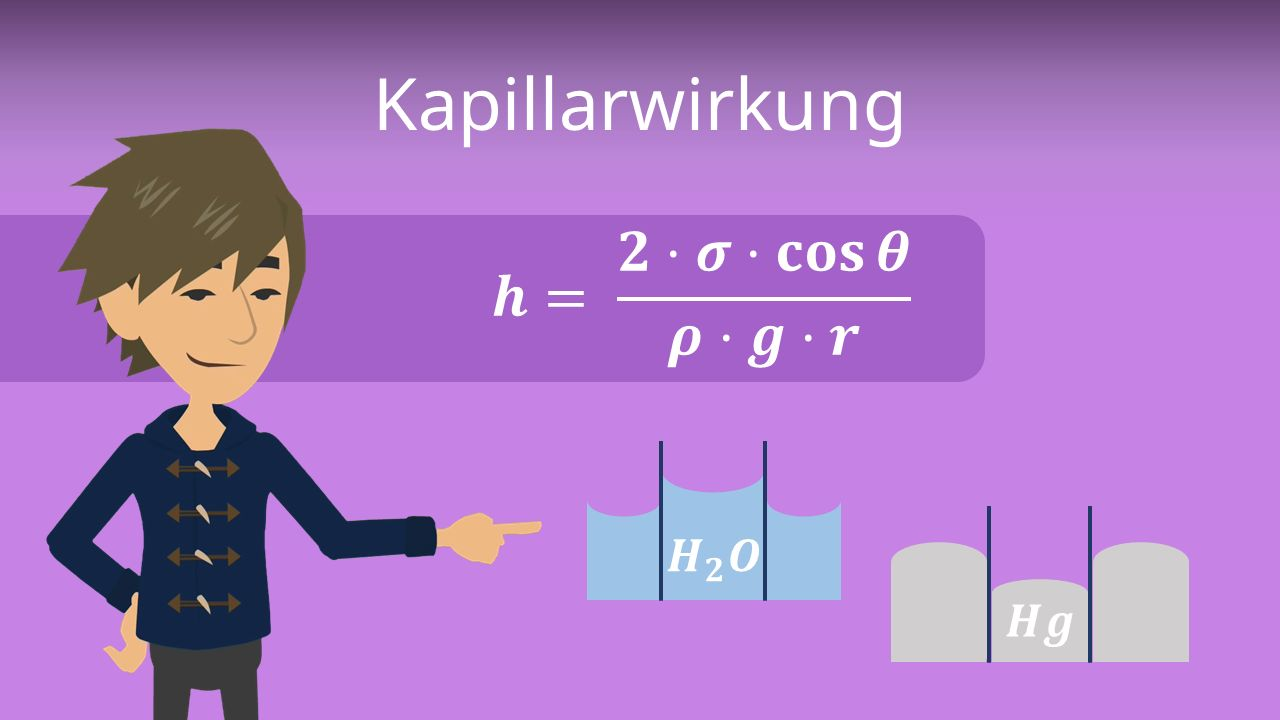CapillaryFormula

h=爬升高度； σ = 表面张力； θ=接触角； ϱ = 密度； g = 重力加速度； r = 半径。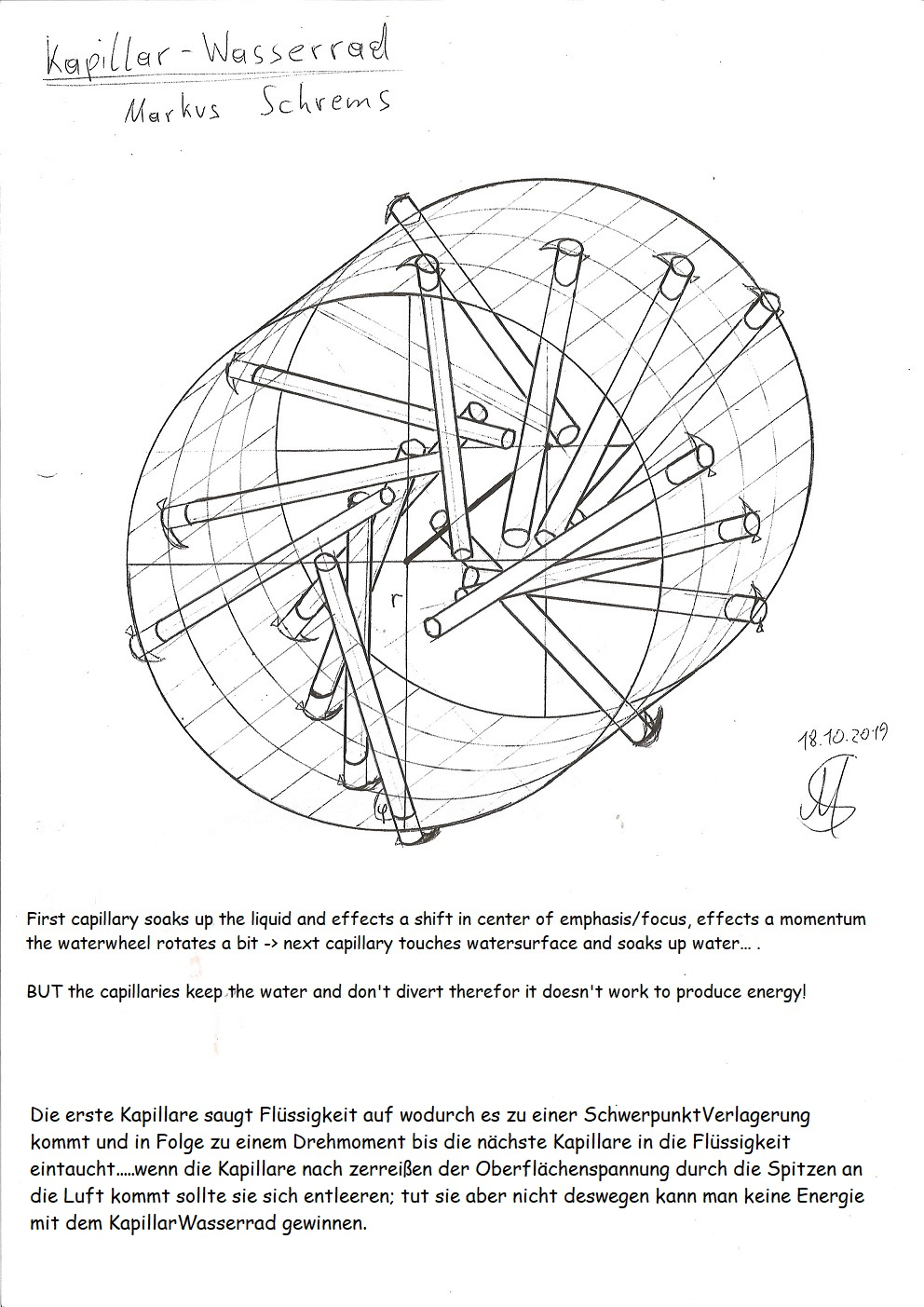Capillary Waterwheel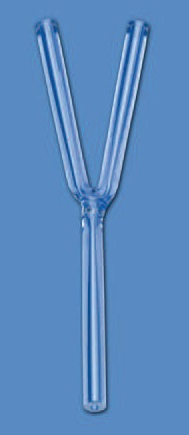YCapillary fills untill both upper ends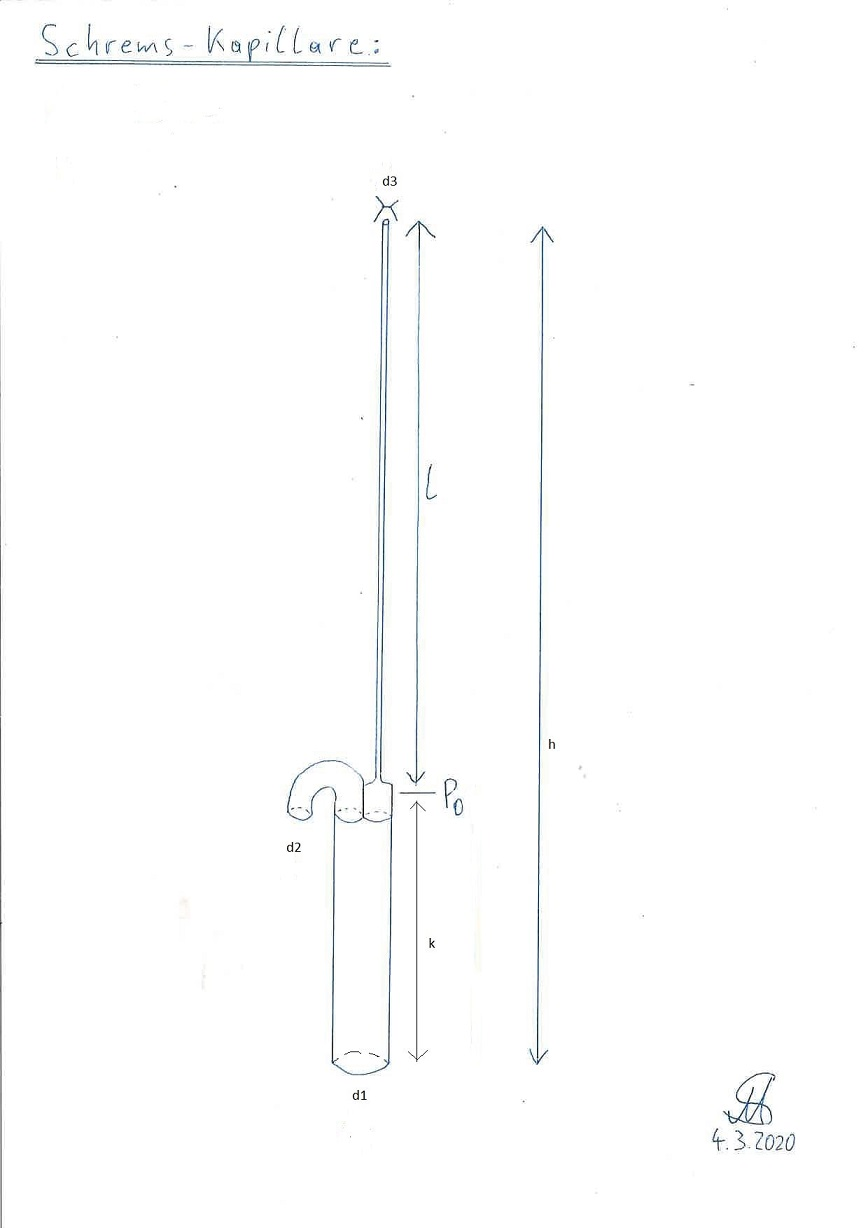Schrems-毛细管：应完全填充自身，但原型的尺寸为：k = 3cm； L=1m； d1 = 0.8mm； d2 = 0.4 mm； d3 = 0,02mm 不起作用。它自行填充，但 d2 处的压力 太低了，所以它没有在那里转移。

## 阀门毛细管 (patent application)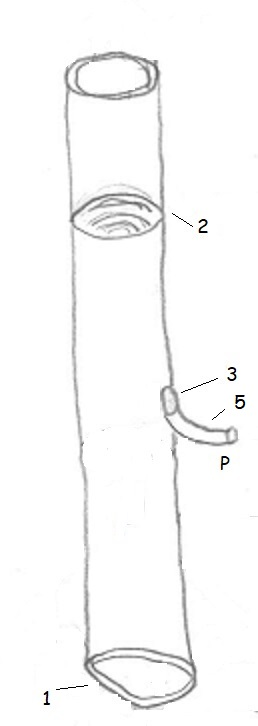## WettingCapillary (patent application)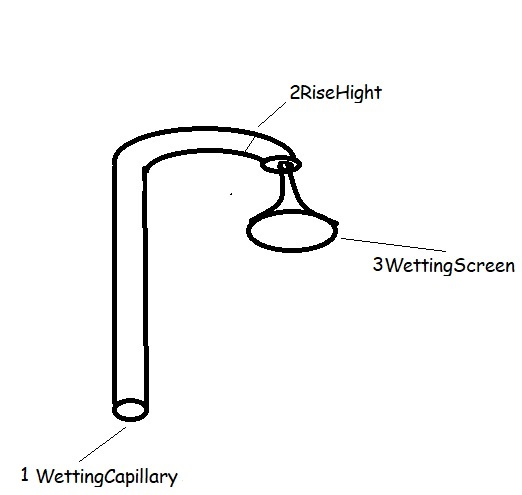## UpliftLift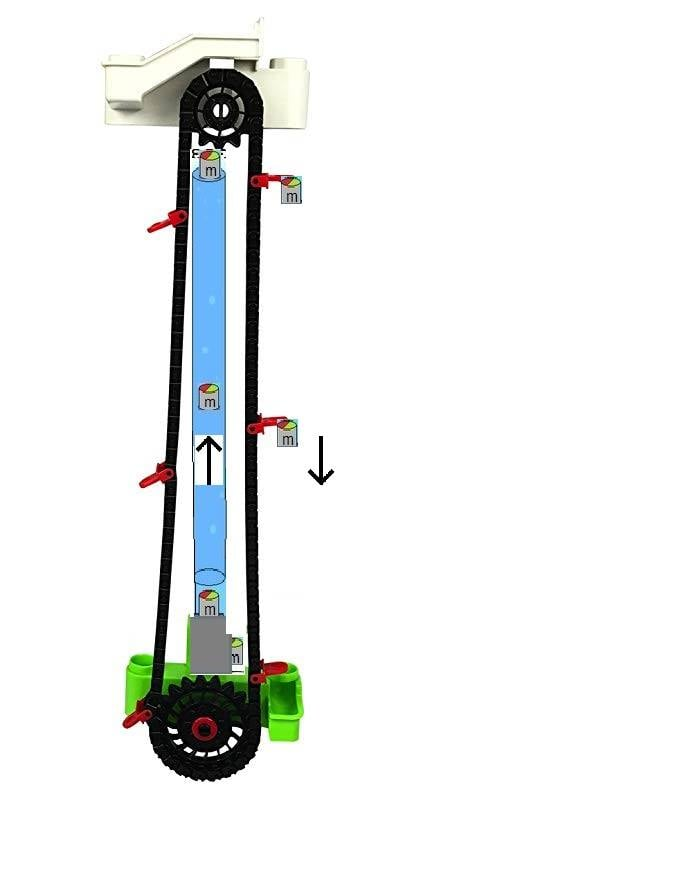UpliftLift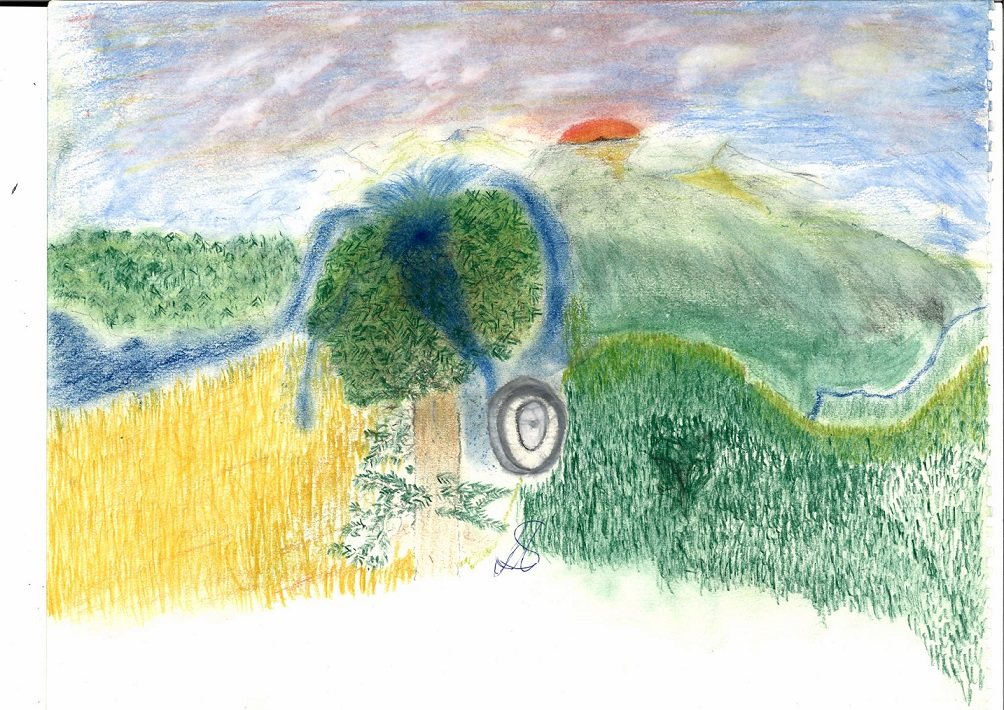SequoyahCapillaryPowerplant

## 荒漠化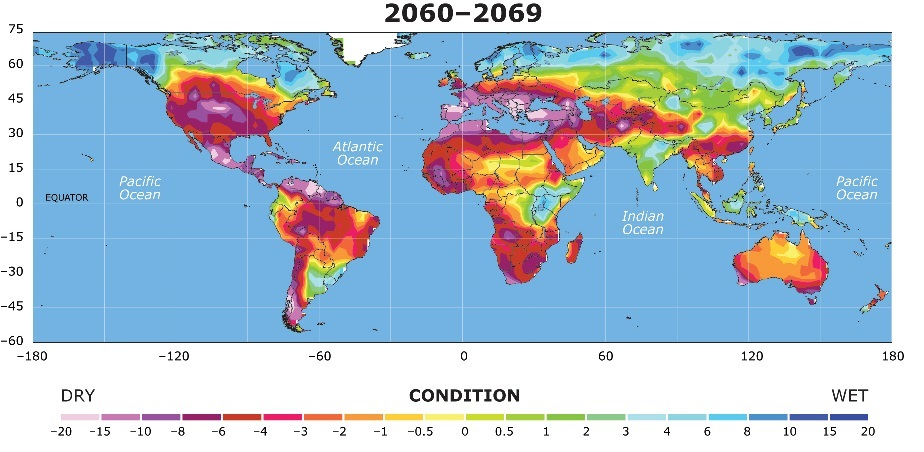## 加速至高速

t = (c/2)/a = (149896229[m/s]) / 59[m/s^2] = 2527232 秒

W = F * d

F = 1000 [kg] * 59.28 [m/s^2]

F = 59280 [N]

d = (1/2) * a * t^2

d = (1/2) * 59 [米/秒^2] * (2527232 [秒])^2

d = 2 * 10^14 [米]

W = F * d

W = 1*10^19 [焦耳]

W / 能量 = 1*10^19 [焦耳] / (4 * 10^15 [焦耳]) = 2500

E_kinetic = (1/2)*m*v²

E_kinetic = (1/2) * 1000 [kg] * (149896229 [m/s])^2

E_kinetic = 1 * 10^19 [焦耳]

...

## 悬浮

ImpulseCover > ImpulseBottom + 加速反冲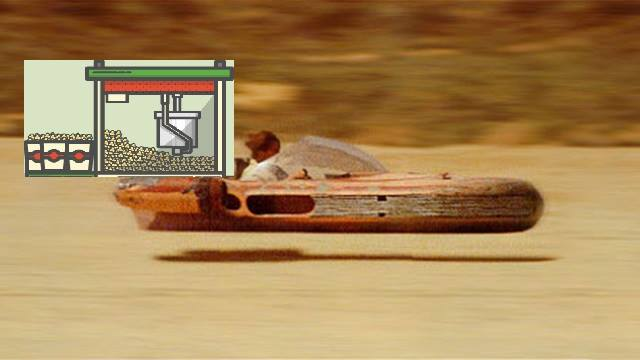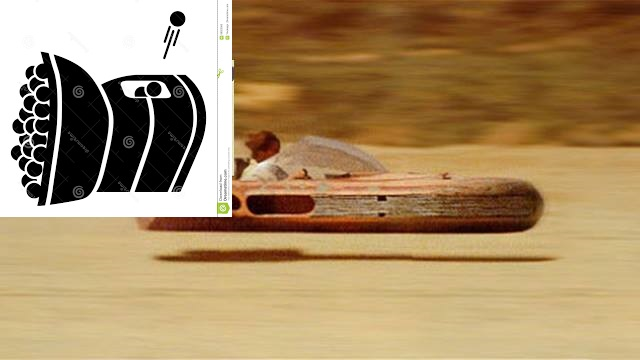Q 是流量（Q = V/t，单位：m³/s）

ρ 是密度 (kg/m3)

V 是体积（立方米）

m 是质量（单位：kg）

g 是重力加速度 (9.8 m/s2)

v 是速度（以米/秒为单位）

t 是时间（以秒为单位）

F_g = (Δv*m)/dt = (Δv*V*ρ)/dt = Δv*Q*ρ = Δv*Q*γ/g = Δv*ṁ

Δv 是盖子处的脉冲速度与底部的脉冲速度之差

γ 比重（N/m3） = kg/(m2s2)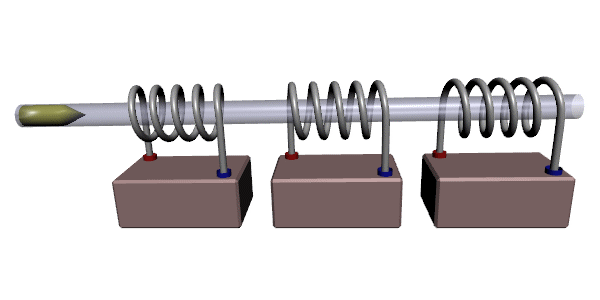线圈枪

v_end = ((2*V* μ_0*χ_m*n²*I²)/m)^1/2

m 是弹丸的质量

V 是射弹体积

χ_m 是磁化率

μ_0 为真空磁导率 4π × 10−7 V*s/(A*m)

I 是电流，单位为安培

n 是线圈单位长度的线圈匝数，通过除以得到 线圈的总匝数可以通过线圈的总长度（以米为单位）来确定

F = (0.5 * B^2 * A * n * l * I^2) / d

B 是线圈枪内的磁场强度（特斯拉）

A为线圈截面积（平方米）

n 是线圈的匝数

l 是线圈的长度（米）

I 是流过线圈的电流（安培）

d 是施加力的距离（米）